# Physics and zeros of the zeta-function

The local statistics of the imaginary parts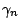of the complex zeros of the Riemann zeta function show the characteristic distribution seen in the eigenvalues of a matrix pulled at random from the unitary group endowed with Haar measure. This collection of matrices is called by physicists the CUE: circular unitary ensemble. For more details on the statistics of the zeros of the zeta function and other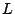-functions, see [L-functions and Random Matrix Theory].

These very same CUE statistics are also seen on a local scale when one studies the distribution of the semiclassical eigenvalues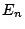of quantum systems having classical analogues that display chaotic behaviour and are not symmetric under time-reversal.

This suggests that thecan be construed as the eigenvalues of some Hermitean operator which is itself obtained by quantizing a classical dynamical system sharing the properties mentioned above: chaoticity and no time-reversal symmetry.

Furthermore, the long-range statistics offor the Riemann zeta function depend on the prime numbers in a manner which is very accurately predicted by formulae with analogues in semiclassical periodic orbit theory. This suggests that the periodic orbits of the hypothetical system underlying the Riemann zeta function would be determined by the positions of the prime numbers.

Clearly the identification of a dynamical system which when quantized produced a Hermitean operator with eigenvaluesrelated to the complex Riemann zeros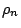by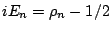would lead to a proof of the Riemann Hypothesis. In the field of quantum chaos studies are made of the very systems which are relevant in such a search, and results from this field suggest what many of the characteristics of such a system should be. For a detailed review of these issues and further references see Berry and Keating [ MR 2000f:11107].

Back to the main index for The Riemann Hypothesis.